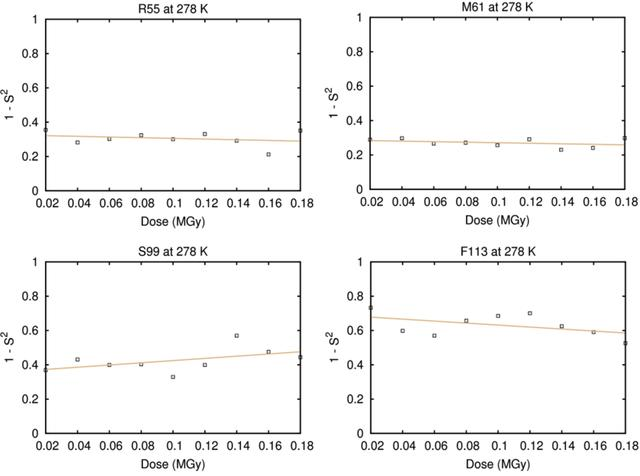disable zoom     view article Figure 5 Order parameters as a function of DWD for the CypA residues R55 (slope = −0.199; p = 0.513), M61 (slope = −0.153; p = 0.373), S99 (slope = 0.643; p = 0.161) and F113 (slope = −0.579; p = 0.201) at 278 K.